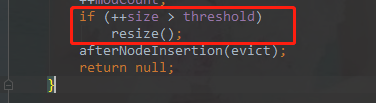# HashMap容量和负载因子

HashMap底层数据结构是数组+链表，JDK1.8中还引入了红黑树，当链表长度超过8个时，会将链表转成红黑树，以提升其查找性能。那么，给出一个<key, value>节点，HashMap是如何确定这个节点应该放在具体哪个位置呢？（以JDK1.8为例）

final V putVal(int hash, K key, V value, boolean onlyIfAbsent,
boolean evict) {
Node<K,V>[] tab; Node<K,V> p; int n, i;
// HashMap没有被初始化，则先进行初始化
if ((tab = table) == null || (n = tab.length) == 0)
n = (tab = resize()).length;
// 节点所在index = (n - 1) & hash，该位置没有数据，则直接将新节点放在数组的index位置上
if ((p = tab[i = (n - 1) & hash]) == null)
tab[i] = newNode(hash, key, value, null);
else { // index上已经有节点了
Node<K,V> e; K k;
// 如果新key与原来的key一样，则e指向原节点p（后面会用新value替换e所指向的value）
if (p.hash == hash &&
((k = p.key) == key || (key != null && key.equals(k))))
e = p;
// 如果该节点是树节点，则采用树的插入算法，插入新节点
else if (p instanceof HashMap.TreeNode)
e = ((TreeNode<K,V>)p).putTreeVal(this, tab, hash, key, value);
else { // 该节点是链表节点
for (int binCount = 0; ; ++binCount) {
// 将新节点插入到index所在链表的末端
if ((e = p.next) == null) {
p.next = newNode(hash, key, value, null);
// 链表节点超过8个，则进行链表转树处理
if (binCount >= TREEIFY_THRESHOLD - 1) // -1 for 1st
treeifyBin(tab, hash);
break;
}
// 同样的，如果key已经存在的话，则不进行插入操作，而是后面进行value替换
if (e.hash == hash &&
((k = e.key) == key || (key != null && key.equals(k))))
break;
p = e;
}
}
// e != null的情况，就是key已经存在了，这里统一进行了新值value，替换旧值e.value的操作
if (e != null) { // existing mapping for key
V oldValue = e.value;
if (!onlyIfAbsent || oldValue == null)
e.value = value;
afterNodeAccess(e);
return oldValue;
}
}
++modCount;
// 插入后数组size 大于阈值的话，需要进行扩容
if (++size > threshold)
resize();
afterNodeInsertion(evict);
return null;
}1、为什么初始容量是16

2、为什么负载因子是0.7503-31244
08-121万+
07-06169
08-126478
05-091257
06-084505
06-18270
06-199291
08-183143
03-26181
08-142万+
07-11380
03-06107
09-103677
11-08349
05-12812
©️2020 CSDN 皮肤主题: 精致技术 设计师:CSDN官方博客